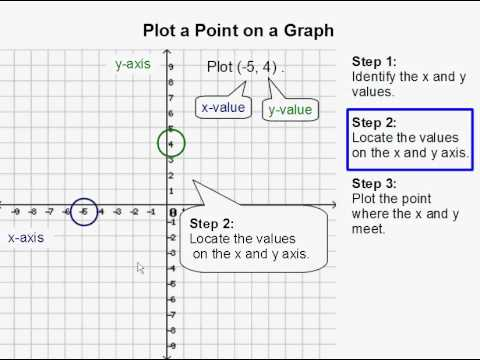3 years ago
reedce
Save
Edit
Host a game
Live GameLive
Homework
Solo Practice
Practice20 QuestionsShow answers
• Question 1
60 seconds
Q. If you were to plot the point (-2, -6) what would be the correct method?
Down 2 , Left 6
Left 6, Down 2
Left 2, Down 6
Left 2, Up 6
• Question 2
60 seconds
Q. If you were to plot the point (4, -12) what would be the correct method?
Up 4, left 12
Right 4, up 12
Down 4, left 12
Right 4, down 12
• Question 3
120 secondsQ. What error did the student make when plotting the point (3, 4) according to the picture?
The student moved left along the x-axis when he should have moved right
The student plotted the y-coordinate in place of the x-coordinate
The student didn't make a mistake
Both Options 1 and 2
• Question 4
30 seconds
Q. The coordinates of a point are (y, x)
True
False
• Question 5
30 seconds
Q. The origin is where the x-axis and y-axis intersect
True
False
• Question 6
60 seconds
Q. Which point is NOT like the rest?
(-3, -5)
(-2, -2)
(1, 7)
(-6, -9)
• Question 7
60 seconds
Q. Which point is NOT like the rest?
(-3, 5)
(6, -2)
(-1, 7)
(-8, 9)
• Question 8
180 seconds
Q. What is true about points (7, -3) and (7, 14)?
They are on the same horizontal line
They are on the same vertical line
They both have positive y-values
They are both located on the x-axis
• Question 9
180 secondsQ. Which letter matches the coordinate (4, -6)?
G
Z
K
None of the above
• Question 10
180 secondsQ. Which letter matches the coordinate (0, -6)?
O
L
i
Z
• Question 11
180 secondsQ. What letter is at (7, -6)?
D
L
X
There is no letter at those coordinates.
• Question 12
180 secondsQ. What letter is at (1,-7 )
There is no letter at those coordinates.
B
C
K
• Question 13
180 secondsQ. What letter is at (3, 8)
There is no letter at those coordinates.
W
R
E
• Question 14
180 secondsQ. What letter is at (-3,7)
There is no letter at those coordinates.
T
E
H
• Question 15
180 secondsQ. What letter is at (-8,-3)
There is no letter at those coordinates.
S
X
R
• Question 16
180 secondsQ. What are the coordinates for point B
(-9,1)
(1,9)
(-9,-1)
(-1,-9)
• Question 17
180 secondsQ. What are the coordinates for point N
(7,9)
(-7,7)
(-9,-7)
(7, 7)
• Question 18
180 secondsQ. What letter is at (7,7)
There is no letter at those coordinates.
L
D
X
• Question 19
180 secondsQ. Which of the following points have a positive y-value?
J, Y, E, Q, S
P, M, G, U
P, L, N, E
L, H, N, O
• Question 20
60 seconds
Q. If you plotted the point (0, -7), you would be __________.